# 1 Introduction

RNA sequencing (RNA-seq) has been utilized as the standard technology for measuring the expression abundance of genes, transcripts, exons or splicing junctions. Numerous quantification methods were proposed to quantify such abundances with/without combination of RNA-seq read aligners. It is currently difficult to evaluate the performance of the best method, due in part to the high costs of running assessment experiments as well as the computational requirements of running these algorithms. We have developed a series of statistical summaries and data visualization techniques to evaluate the performance of transcript quantification.

The rnaseqcomp R-package performs comparisons and provides direct plots on these statistical summaries. It requires the inputs as a list of quantification tables representing quantifications from compared pipelines on a two condition dataset. With necessary meta information on these pipelines (e.g. names) and annotation information for quantified features (e.g. transcript information), a two step analysis will generate the desired evaluations.

1. Data filtering and data calibration. In this step, options are provided for any filtering and calibration operations on the raw data. A S4 class rnaseqcomp object will be generated for next step.

2. Statistical summary evaluation and visualization. Functions are provided for specificity and sensitivity evaluations.

# 2 Getting Started

library(rnaseqcomp)

# 3 Preparing Data

For each compared pipeline, a quantification table should be a $$m*n$$ matrix, where $$m$$ corresponding to the number of quantified features (e.g. transcripts) and $$n$$ corresponding to the number of samples. The function signalCalibrate takes a list of these matrices as one of the inputs, with extra options such as meta information of pipelines, features for evaluation and features for calibration, and returns a S4 rnaseqcomp object that contains everything for downstream evaluation.

There are several reasons why we need extra options in this step:

1. Meta information of pipelines basically are factors to check the sanity of table columns, and to provide unique names of pipelines for downstream analysis.

2. Since there might be dramatic quantification difference between different features, e.g. between protein coding genes and lincRNA genes, evaluations based on a subset of features can provide stronger robustness than using all involved features. Thus, an option is offered for selecting subset of features.

3. Due to different pipelines might report different units of quantification, such as FPKM (fragments per kilobases per million), RPKM (reads per kilobases per million), TPM (transcripts per million) etc. Calibrations across different pipelines are necessary. Options are provided in the way that on which features the calibrations are based and to what pipeline the signals are mapped.

4. Annotations for features basically provide the differential expression status and meta relationships between different kinds of features, such as which transcripts belong to which genes.

We show here an example of selecting house-keeping genes(Eisenberg and Levanon 2013) on chromosome 1 for calibration and using all transcripts for evaluation. In this vignette, we will use enbedded dataset simdata as one example to illustrate this package.

This dataset include quantifications on 15776 transcripts on two simulated cell lines each with 8 replicates. The true differential expressed transcripts were simulated. Illustration quantifications from two pipelines (RSEM(Li and Dewey 2011) and FluxCapacitor(Montgomery et al. 2010)) are included in this dataset.

# load the dataset in this package
data(simdata)
class(simdata)
##  "list"
names(simdata)
##  "quant" "meta"  "samp"

Here, quantifications are included in simdata$quant. Meta information of transcripts is included in simdata$meta, inlcuding if they belongs to house keeping genes and their simulated true fold change status. Sample information is included at simdata$samp. In order to fit into function signalCalibrate, necessary transformation to factors or logical vectors are needed for extra options as shown below. condInfo <- factor(simdata$samp$condition) repInfo <- factor(simdata$samp$replicate) evaluationFeature <- rep(TRUE, nrow(simdata$meta))
calibrationFeature <- simdata$meta$house & simdata$meta$chr == 'chr1'
unitReference <- 1

The return value of signalCalibrate is a S4 rnaseqcomp object, of which general information can be viewed by generic function show.

dat <- signalCalibrate(simdata$quant, condInfo, repInfo, evaluationFeature, calibrationFeature, unitReference, calibrationFeature2 = calibrationFeature) class(dat) ##  "rnaseqcomp" ## attr(,"package") ##  "rnaseqcomp" show(dat) ## rnaseqcomp: Benchmarks for RNA-seq quantification pipelines ## ## Quantifications pipelins: 2 ## Total transcripts: 15776 ## Total samples from 2 conditions: 16 # 4 Visualizing Benchmarks Five type of QC metrics can be evaluated by this package currently. Please refer to our paper for more details(Teng et al. 2016). ## 4.1 Specificity on expressed features. This metric is evaluated by the quantification deviations between RNA-seq technical replicates. Basically lower deviations indicate higher specificity. Both one number statistics and graphes of standard deviations stratified by expression signals are provided. Specifically, the one number statistics are summarized separately based on three different levels of expression signals, as standard deviation does change dramatically with different levels of expression. plotSD(dat,ylim=c(0,1.4)) ##$med
##       rsem fluxcapacitor
## A<=1  0.65          0.99
## 1<A<6 0.42          1.10
## A>=6  0.21          0.32
##
## $se ## rsem fluxcapacitor ## A<=1 0.003 0.005 ## 1<A<6 0.003 0.008 ## A>=6 0.001 0.002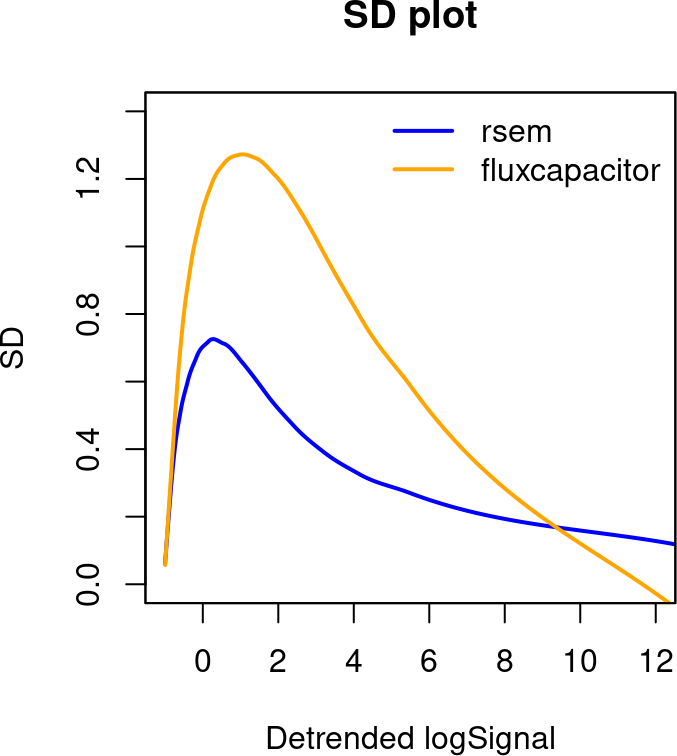Detrended signals shown in the plot are actually the signals with the same scales as RSEM pipeline, as we selected this pipeline as unitReference. In this case, TPM by RSEM. In the returned matrix, values are based on average of two cell lines; the “A” in row names means the detrended log signals. Basicallly, this figure shows RSEM quantification has lower standard deviation than FluxCapacitor. ## 4.2 Specificity on non-expressed features The proportions of non-expressed features is another important statistics. Two types of non-expressed features are analyzed simultaneously: ### 4.2.1 Features expressed in one technical replicate but not the other. Given a cutoff to define if one signal indicating express or non-express, a proportion of transcripts might express in one replicate but not the other in any compared two replicates. Thus, a lower proportion of such transcripts indicates a better specificity. We calculate the average of proportions from each two-replicate comparison as we have more than two replicates in each cell line. ### 4.2.2 Features expressed in neither replicates. Using the same cutoffs as above, a proportion of transcripts might express in neither of compared replicates. This metric should be analyzed jointly with the metric above. For more details, please refer to our paper(Teng et al. 2016). plotNE(dat,xlim=c(0.5,1)) ##$NE
##    rsem fluxcapacitor
## 0 0.097         0.163
## 1 0.073         0.145
## 2 0.049         0.120
## 3 0.034         0.095
##
## $NN ## rsem fluxcapacitor ## 0 0.615 0.559 ## 1 0.692 0.629 ## 2 0.768 0.704 ## 3 0.832 0.775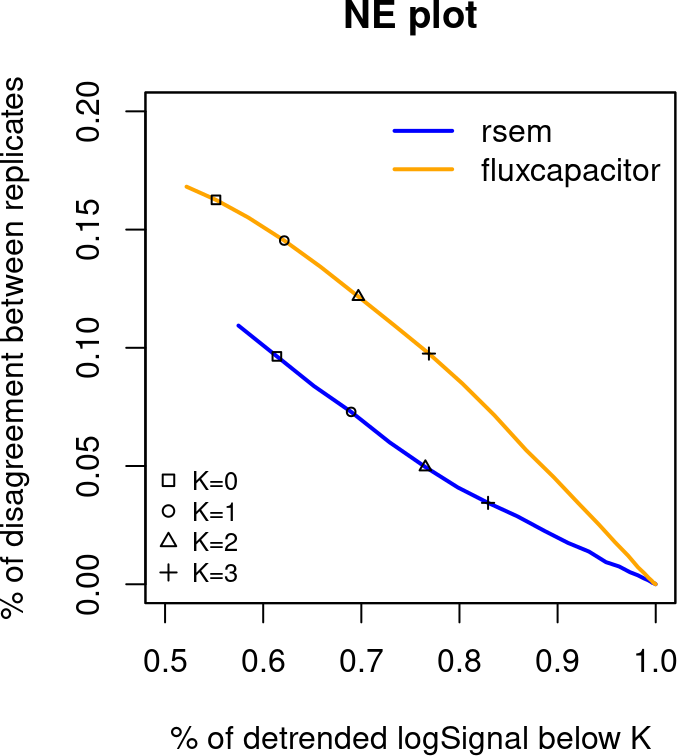Here, y axis indicates express and non-express proportion, and x-axis indicates both non-express proportion. Again, the returned values are based on average of two cell lines, while “NE” matrix represents express and non-express proportions and “NN” matrix represents both non-express proportions. For row names of returned matrices above, 0,1,2,3 indicate corresponding cutoffs. ## 4.3 Specificity for genes only have two annotated transcripts For any compared two replicates in each cell line, the proportion of one transcript for genes that only include two annotated transcripts can be different even flipped. This section estimates and plots the proportion difference stratefied by detrended logsignal. Averages of absolute difference will be reported for three levels of detrened logsignals. plot2TX(dat,genes=simdata$meta$gene,ylim=c(0,0.6)) ##$mean
##       rsem fluxcapacitor
## A<=1  0.41          0.44
## 1<A<6 0.07          0.14
## A>=6  0.04          0.07
##
## $se ## rsem fluxcapacitor ## A<=1 0.014 0.016 ## 1<A<6 0.004 0.006 ## A>=6 0.003 0.004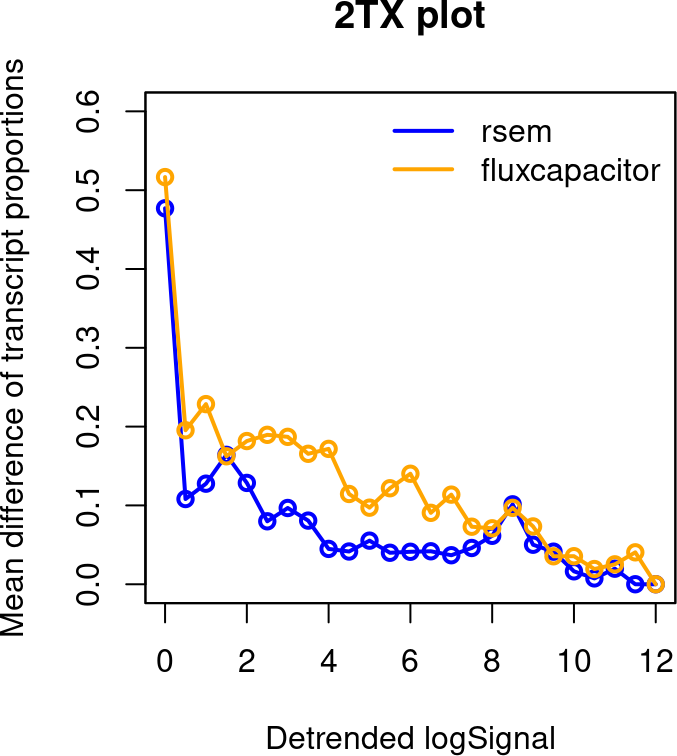Basically higher curve indicates worse specificity for expression of genes that only have two transcripts. The returned matrix is based on three different levels of detrended logsignals. Similar explanation can be found as plotSD. ## 4.4 Sensitivity in differential analysis ### 4.4.1 ROC curves For each pipeline, differential expression is first estimated by fold change on 1 vs. 1 comparison between cell lines. ROC curves then are made by comparing fold changes with predefined true differentials. ROC curves from multiple 1 vs. 1 comparisons are averaged using threshold averaging strategy. Standardized partial area under the curve (pAUC) is reported for each pipeline. plotROC(dat,simdata$meta$positive,simdata$meta$fcsign,ylim=c(0,0.8)) ## rsem fluxcapacitor ## 0.630 0.512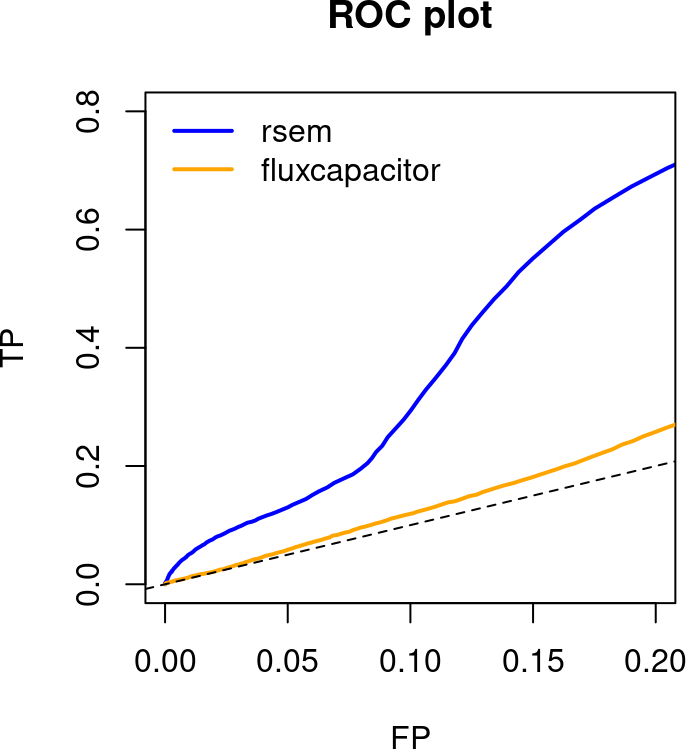### 4.4.2 Distribution of estimated fold changes For each pipeline, differential expression is estimated by fold change on mean signals across replicates of cell lines. For features that are truely differential expressed, their fold changes levels are summarized based on different levels of detrended logsignals. simdata$meta$fcsign[simdata$meta$fcstatus == "off.on"] <- NA plotFC(dat,simdata$meta$positive,simdata$meta$fcsign,ylim=c(0,1.2)) ##$med
##       rsem fluxcapacitor
## A<=1  0.53          0.39
## 1<A<6 0.96          0.77
## A>=6  0.93          0.85
##
## \$se
##        rsem fluxcapacitor
## A<=1  0.012         0.016
## 1<A<6 0.012         0.021
## A>=6  0.007         0.013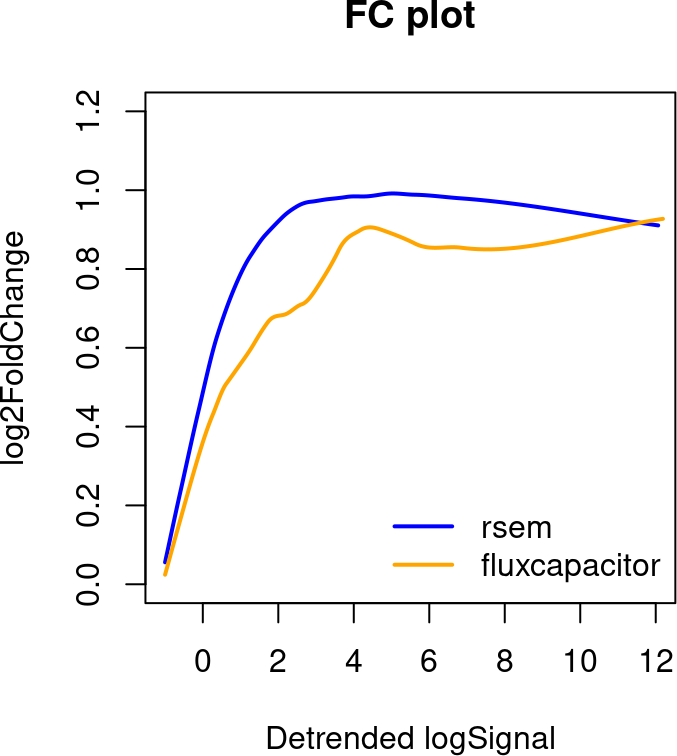Here, in the embeded simulated data. Several transcripts are simulated as on and off pattern, meaning expressed in one cell line and no signal at all in the other. Those transcripts might bias the true distribution we want, since their fold change could be infinity. So we ignored those transcripts by setting their true signs of fold changes to NA before running the function “plotFC”.

# 5 Summary

In this vignette, we basically go through the major functions included in this package, and try to illustrate how they work. The data used is actually partial of the data as we shown in our paper. We demonstrate that by combining these metrics together, one can easily get how their running pipeline performances are and make further decisions baed on that.